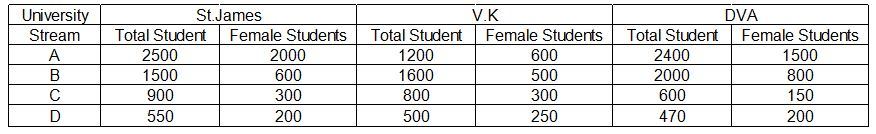## SBI PO Quant Test 23

Instructions

Study the table and answer the given questions
Data regarding number of students studying in various streams in various universities (St. James, V. K. and DVA) in the year 2009Question 1

What is the respective ratio between the total number of females studying in stream C in all the universities together and the total number of females studying in stream D in all the universities together ?

Question 2

Total number of males studying in stream A in all the universities together in 2010 is 1200 more than that in the year 2009. In 2010, what was the total number of students (male + female) studying in stream A in all the universities together, if the total number of male students in stream A in 2010, constituted 50% of the total number of students ?

Question 3

Total number of students studying in streams A and B together in V.K. are what percent less than those studying in same streams together in St. James ?

Question 4

What is the average number of male students studying in stream D in all the given universities ?

Question 5

Number of students studying in stream C in V.K. is what percent of that studying in stream B in DVA ?